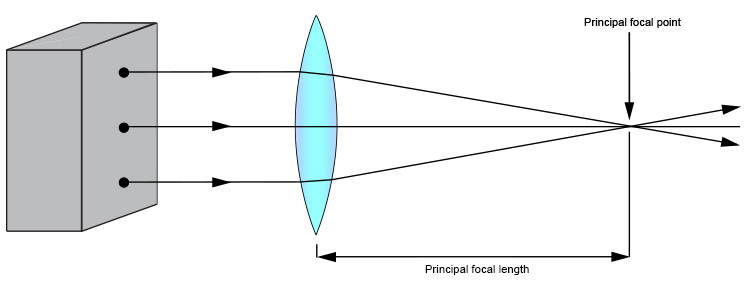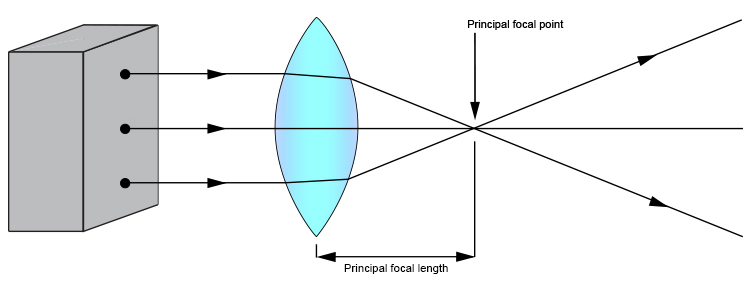# Practical ways to find the principal focal length – lightbox

The simplest way to find the principal focal length of a convex lens is to use a lightbox with three parallel beams.Pass the three beams through the convex lens and you can clearly see the principal focal point. (NOTE: Normally the light box has slits of light so that you can trace the light rays on the background board.)

Then you can work out the principal focal length by measuring between the centre of the lens and the principal focal point.

If you use a convex lens of greater curvature, you will find the angles coming off the lens to be steeper and so the principal focal length will be shorter.